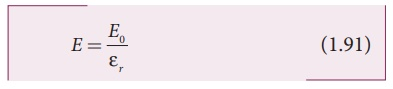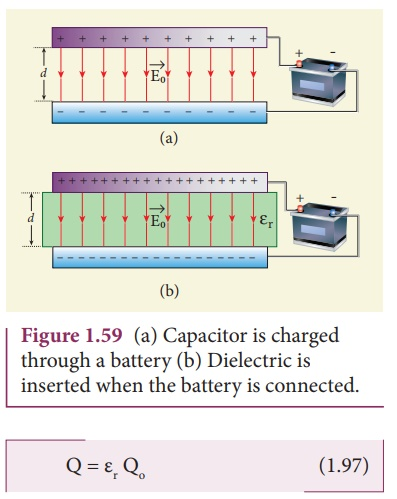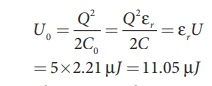Home | | Physics 12th Std | Effect of dielectrics in capacitors

# Effect of dielectrics in capacitors

The dielectric can be inserted into the plates in two different ways. (i) when the capacitor is disconnected from the battery. (ii) when the capacitor is connected to the battery.

Effect of dielectrics in capacitors

In earlier discussions, we assumed that the space between the parallel plates of a capacitor is either empty or filled with air. Suppose dielectrics like mica, glass or paper are introduced between the plates, then the capacitance of the capacitor is altered. The dielectric can be inserted into the plates in two different ways. (i) when the capacitor is disconnected from the battery. (ii) when the capacitor is connected to the battery.

## (i) when the capacitor is disconnected from the battery

Consider a capacitor with two parallel plates each of cross-sectional area A and are separated by a distance d. The capacitor is charged by a battery of voltage V0 and the charge stored is Q0. The capacitance of the capacitor without the dielectric isThe battery is then disconnected from the capacitor and the dielectric is inserted between the plates. This is shown in Figure 1.58.The introduction of dielectric between the plates will decrease the electric field. Experimentally it is found that the modified electric field is given byHere Eo is the electric field inside the capacitors when there is no dielectric and εr is the relative permeability of the dielectric or simply known as the dielectric constant. Since εr > 1, the electric field E < Eo.

As a result, the electrostatic potential difference between the plates (V = Ed) is also reduced. But at the same time, the charge Qo will remain constant once the battery is disconnected.

Hence the new potential difference isWe know that capacitance is inversely proportional to the potential difference. Therefore as V decreases, C increases.

Thus new capacitance in the presence of a dielectric isSince εr > 1, we have C > Co. Thus insertion of the dielectric constant εr increases the capacitance.

Using equation (1.84),where ε = εr εo is the permittivity of the dielectric medium.

The energy stored in the capacitor before the insertion of a dielectric is given byAfter the dielectric is inserted, the charge Q0 remains constant but the capacitance is increased. As a result, the stored energy is decreased.Since εr > 1 we get U < Uo. There is a decrease in energy because,when the dielectric is inserted, the capacitor spends some energy in pulling the dielectric inside.

## (ii) When the battery remains connected to the capacitor

Let us now consider what happens when the battery of voltage V0 remains connected to the capacitor when the dielectric is inserted into the capacitor. This is shown in Figure1.59.

The potential difference V0 across the plates remains constant. But it is found experimentally (first shown by Faraday) that when dielectric is inserted, the charge stored in the capacitor is increased by a factor εr.Due to this increased charge, the capacitance is also increased. The new capacitance isHowever the reason for the increase in capacitance in this case when the battery remains connected is different from the case when the battery is disconnected before introducing the dielectric.The energy stored in the capacitor before the insertion of a dielectric is given byNote that here we have not used the expressionbecause here, both charge and capacitance are changed, whereas in equation (1.100), Vo remains constant.

After the dielectric is inserted, the capacitance is increased; hence the stored energy is also increased.Since εr > 1 we have U > Uo.

It may be noted here that since voltage between the capacitor V0 is constant, the electric field between the plates also remains constant.

The energy density is given bywhere ε is the permittivity of the given dielectric material.

The results of the above discussions are summarised in the following Table 1.2EXAMPLE 1.21

A parallel plate capacitor filled with mica having εr = 5 is connected to a 10 V battery. The area of the parallel plate is 6 m2 and separation distance is 6 mm.

(a) Find the capacitance and stored charge.

(b) After the capacitor is fully charged, the battery is disconnected and the dielectric is removed carefully.

Calculate the new values of capacitance, stored energy and charge.

Solution

(a) The capacitance of the capacitor in the presence of dielectric is(b) After the removal of the dielectric, since the battery is already disconnected the total charge will not change. But the potential difference between the plates increases. As a result, the capacitance is decreased.

New capacitance isThe stored charge remains same and 442.5 nC. Hence newly stored energy isThe increased energy is

∆U = 11.05µ J −2.21 µ J = 8.84 µ J

When the dielectric is removed, it experiences an inward pulling force due to the plates. To remove the dielectric, an external agency has to do work on the dielectric which is stored as additional energy. This is the source for the extra energy 8.84 µJ.

Study Material, Lecturing Notes, Assignment, Reference, Wiki description explanation, brief detail
12th Physics : Electrostatics : Effect of dielectrics in capacitors |